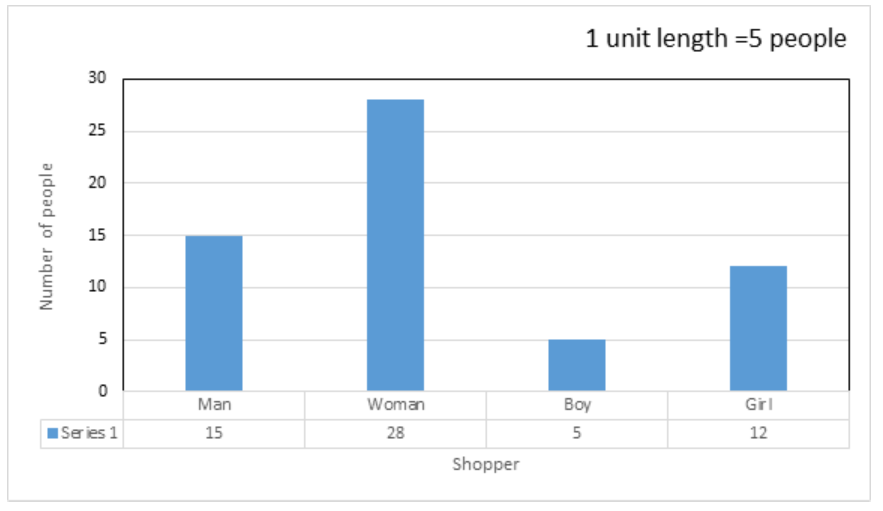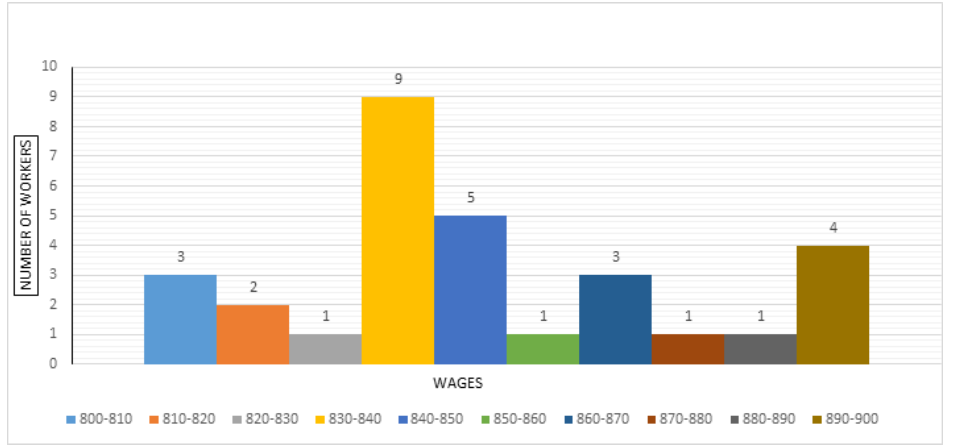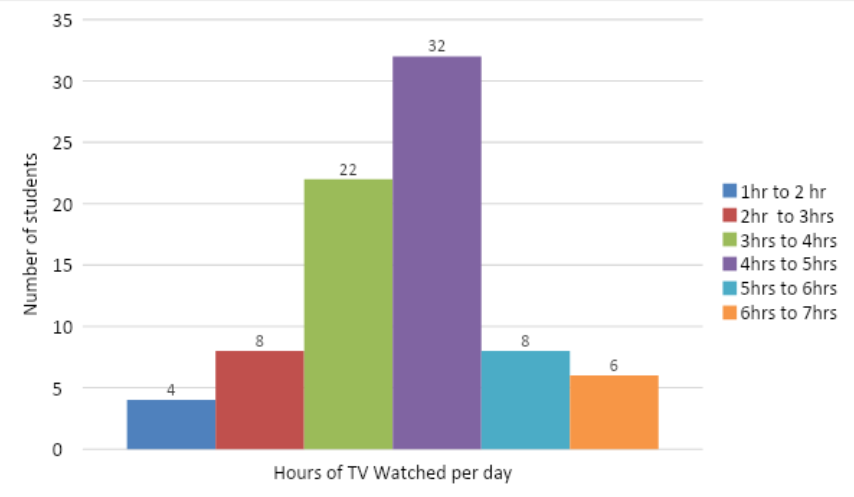Courses
Courses for Kids
Free study material
Free LIVE classes
More

# NCERT Solutions for Class 8 Maths Chapter 5 Data Handling (EX 5.1) Exercise 5.1## NCERT Solutions for Class 8 Maths Chapter 5 Data Handling (EX 5.1) Exercise 5.1

NCERT Solutions for Class 8 Maths Chapter 5 are provided by Vedantu to simplify their exam preparation. Drafted by subject experts, our solutions for Class 8 Maths Chapter 5 Exercise 5.1 are deemed the most preferred for exams. Also, our NCERT Solutions for Class 8 Maths Chapter 5 Exercise 5.1 have the shortcut techniques as well as step-by-step methods to help you gain an in-depth knowledge of the same. You can download NCERT Solutions PDF to boost your scores in your upcoming exam. Science Students who are looking for NCERT Solutions for Class 8 Science will also find the Solutions curated by our Master Teachers really Helpful.

Last updated date: 30th May 2023
Total views: 474k
Views today: 11.31k

## Access NCERT Solutions for Class 8 Chapter 5 - Data Handling

Exercise 5.1

Refer to page 3 for Exercise 5.1 in the PDF

1. For which of these would you use a histogram to show the data.

(a) The number of letters for different areas in a postman’s bag.

Ans: In this part we have to check that we can use histogram or not to represent the number of letters for different areas in a postman’s bag. So, it is clear that we cannot represent that type of data in the form of a histogram. It is because to represent the data in the form of histogram we need class intervals but in this part classes are the names of different places and the term class interval is meaningless in this type of data. So, we cannot use histogram to represent the number of letters for different areas in a postman’s bag.

(b) The height of competitors in an athletics meet.

Ans: In this part we have to check that we can use histogram or not to represent the height of competitors in an athletics meet. So, we can use a histogram to represent such a type of data as the given data is continuous. We can calculate the class interval by subtracting the lower class limit from the upper class limit.

(c) The number of Cassettes produced by 5 companies.

Ans: In this part we have to check that we can use histogram or not to represent the number of Cassettes produced by 5 companies. Again in this type of data we cannot use histogram to represent data. Because we cannot calculate the class interval because the classes in this problem are the name of the companies and the class interval is meaningless in this problem as we cannot find the numerical width of any class.

(d) The number of passengers boarding trains from 7.00 a.m. to 7.00 p.m. at a station.

Ans: In this part we have to check whether we can use histogram or not to represent the number of passengers boarding trains from 7.00 a.m. to 7.00 p.m. at a station. Since in this part we can calculate the class interval, we can use the histogram to represent the number of passengers boarding trains from 7.00 a.m. to 7.00 p.m. at a station.

2. The shoppers who come to a departmental store are marked as: man (M), woman (W), boy (B) or girl (G). The following list gives the shoppers who came during the first hour in the morning. W W W G B W W M G G M M W W W W G B M W B G G M W W M M W W W M W B W G M W W W W G W M M W M W G W M G W M M B G G W. Make a frequency distribution table using tally marks. Draw a bar graph to illustrate it.

Ans: The representation of data by frequency Distribution table using tally marks is as follows:

 Shopper Tally Marks Frequency M(Man) |||||||||||| 15 W(woman) ||||||||||||||||||||||| 28 B(Boy) |||| 5 G(Girl) |||||||| || 12 Total 60

And now the bar graph is:3. The weekly wages (in `) of 30 workers in a factory are: 830, 835, 890, 810, 835, 836, 869, 845, 898, 890, 820, 860, 832, 833, 855, 845, 804, 808, 812, 840, 885, 835, 835, 836, 878, 840, 868, 890, 806, 840. Using tally marks, make a frequency table with intervals as 800 – 810, 810 – 820 and so on.

Ans: The representation of data by frequency distribution table using tally marks is as follows:

 Class Intervals Tally Marks Frequency 800 – 810 ||| 3 810 – 820 || 2 820 – 830 | 1 830 – 840 ||||  |||| 9 840 – 850 |||| 5 850 – 860 | 1 860 – 870 ||| 3 870 – 880 | 1 880 – 890 | 1 890 – 900 |||| 4 Total 30

4. Draw a histogram for the frequency table made for the data in Question 3 and answer the following questions.

Ans: The histogram for the frequency table for the data given in Question 3 is as follows.(i) How many workers earn ` 850 and more?

Ans: In this part we have to calculate how many workers earn 850 and more wages weekly out of 30 workers. So, we can clearly see in both the frequency distribution table and histogram that 10 workers can earn 850 and more wages weekly.

(ii) How many workers earn less than ` 850?

Ans: In this part we have to calculate how many workers earn less than 850 wages weekly.

So, we can find the number of workers who are earning less than 850 wages weekly in three ways.

(a) First by frequency distribution.

In the frequency distribution table it is clearly seen that the number of workers who earn less than 850 wages weekly are 20.

(b) Second by histogram.

Also in histogram it is clearly seen that the number of workers who earn less than 850 wages weekly are 20.

That is, the sum of the number of workers from the class 800 – 810 to 840 – 850.

(c) Third, by subtracting the number of workers who earn 850 wages and more weekly from the total number of workers.

So, we know that

The total number of workers working in a factory is 30.

The number of workers who earn 850 wages or more weekly is 10.

Therefore,

The number of workers how earns less than 850 wages weekly \$ = 30 - 10\$

\$ = 20\$

So, the number of workers earning less than 850 wages weekly is 20.

5. The number of hours for which students of a particular class watched television during holidays is shown through the given graph.

We draw the histogram for above frequency table:(i) For how many hours did the maximum number of students watch T.V.?

Ans: In this part we have to find how many hours did the maximum number of students watch T.V.

So, from the histogram we can clearly see that 32 students watch T.V. for 4 to 5 hours per day.

(ii) How many students watched TV for less than 4 hours?

Ans: In this part we have to find how many students watched T.V. for less than 4 hours.

That is, a number of students watched T.V. 1 to 4 hours per day.

Therefore, from the histogram the number of students who watch T.V. for less than 4 hours \$ = 4 + 8 + 22\$

\$ = 34\$

That is, 34.

(iii) How many students spent more than 5 hours watching TV?

Ans: In this part we have to find the number of students who watched T.V for more than 5 hours per day.

That is, the number of students who watched T.V. 5 to 7 hours per day.

So, from histogram we can find the number of students who watched T.V. for more than 5 hours.

\$8 + 6 = 14\$

So, the number of students who watch T.V. for more than 5 hours is 14.

## NCERT Solutions for Class 8 Maths Chapter 5 Data Handling – Chapter Overview

Data handling plays a significant role for students in learning Maths as it helps them deal with real-life situations. Besides, it also develops a critical-thinking ability in them and tests their reasoning ability. In the case of data handling, students are taught to classify data. In the case of Exercise 5.1 of the chapter, there is a particular emphasis on the following –

• Graphical representation of data via

• Pictograph

• Bar graph

• Double bar graph

• Organising data

• Grouping data

• Frequency distribution table

• Histogram, etc.

While such problems have real-life applications, students need to catch up on the tricks that will help them solve such questions with ease. Consequently, they need a proper understanding of various concepts along with the shortcut techniques to solve the same within a stipulated time frame. With our NCERT Solutions for Class 8 Maths Chapter 5 Data Handling, you can get the most preferred exam guide to help you gain knowledge about it.

### Here is a Brief Explanation of Every Question Included in this Chapter.

• Class 8 Maths Chapter 5 Exercise 5.1 – Question 1

In the first question, you are provided with four statements and asked to determine under which you would need to draw a histogram.

From our NCERT Maths Class 8 Chapter 5 Exercise 5.1, you can learn how to deal with these questions. We have a step by step guide to help you have a clear idea about histograms and also how to draw them.

• Maths Chapter 5 Class 8 Exercise 5.1 – Question 2

The second question gives you raw data out of which you need to find the frequency of each parameter with the use of tally marks. After that, you need to draw a bar graph as well.

In our NCERT Solutions for Class 8 Maths Chapter 5 Data Handling, we have shown a stepwise process with brief explanations of them, for your understanding.

• NCERT Class 8 Maths Chapter 5 Exercise 5.1 – Question 3

At first, question 3 might look similar to the previous one, but you need to identify that the given raw data is asked to be represented in equal intervals.

From our NCERT Solution for Class 8 Maths Chapter 5 Exercise 5.1, you will get to know about the process to frame such tables.

• Class 8 Maths Chapter 5 Exercise 5.1 – Question 4

Question 4 asks you to draw a histogram based on the data given in question 3. Two short questions follow which can be answered post-drawing the histogram. Subsequently, you need to know how to draw the same out of the provided data.

Our NCERT Solutions for Class 8 Maths Chapter 5 Data Handling comes equipped with step by step methods to ensure that students can derive the accurate answer in the shortest possible time. Since professionals draft these solutions to Maths Class 8 Chapter 5 Exercise 5.1, you are likely to receive the top class study notes for your guidance.

• NCERT Class 8 Maths Chapter 5 Exercise 5.1 – Question 5

The last question of the exercise comprises a graph that has

• Number of students

• Number of hours spent watching television

Plotted in the X and Y-axis. While the question has three subparts to it, such questions are the most preferred to be included in the exam papers. Take up our NCERT Solutions for Class 8 Maths Chapter 5 Exercise 5.1 and delve into the details of solving such questions. Let these solutions prepare you well for your upcoming examination.

### Vedantu – Where Learning Knows No Limits!

At Vedantu, we have a group of eminent faculties working relentlessly to transform the imagination into reality - where knowledge meets skills. Our main objective is to provide an interactive platform with profound knowledge for students who aim higher in their career.

We have provided our NCERT Solutions for Class 8 Maths Chapter 5 Data Handling in more than 1000 cities. Over 40,000 students have significantly benefitted from our course materials.

These solutions for Class 8 Maths Chapter 5 Exercise 5.1 are equipped with explanations in the straightforward language. With tables and charts, students have the provision of gaining convenient access to a learning guide sans any charges.

With exams around the corner, how are you still waiting?

Grab our study notes now and become a pro in all your subjects!